# .css-4zleql{display:block;}Anuj Syal's Blog# Word Vectorization: A Revolutionary Approach In NLP

Anuj Syal
·Aug 19, 2020·

Subscribe to my newsletter and never miss my upcoming articles

Photo by Hope House Press — Leather Diary Studio on Unsplash

Language allows humans to communicate their ideas for enhanced understanding. Similarly, in AI and ML, the use of Natural Language Processing (NLP) advances deep learning models for input which can also be non-numerical.

In NLP, a methodology called Word Embeddings or Word Vectorization is used to map words or phrases from vocabulary to a corresponding vector of real numbers to enable word predictions, word similarities/semantics. This process of converting words into numbers is called Vectorization.

## Word Vector…What?

To help those new to AI mumbo-jumbo, I will try to explain it in a simpler manner. Take, for example, you are talking to someone who does not know or understand your language. So you use gestures or objects to explain to them an idea. Word Vectorization can also be understood in the same manner. For deep learning models, comprehending text or words in their original form is not possible. Therefore, Word Vectorization turns individual words into vectors for easy consumption and comprehension by the machine learning algorithm.

### What is a Vector?

Vector denotes the mathematical or geometrical representation quantity. Consider a vector of geometrical point P [2, 3, 4]. This vector basically represents the point P in 3-dimensional space.3 Dimensional vector for point P

### N-dimensional word vector

In NLP consider an n-dimensional word vector in space of n-dimensions. The dimension can vary from 10 to 1000. Where each word would have from 1- -1000 coordinates. Visualizing this would be difficult but you can just consider this as an extension of the 3d vectors where the coordinates are increased drastically. This placing each word in n-dimensional space.Image of Tesseract from Interstellar showing multiple dimensions

As an example, after running a word vectorization across a large text 7-dimensional word vector for `banking` looks like this.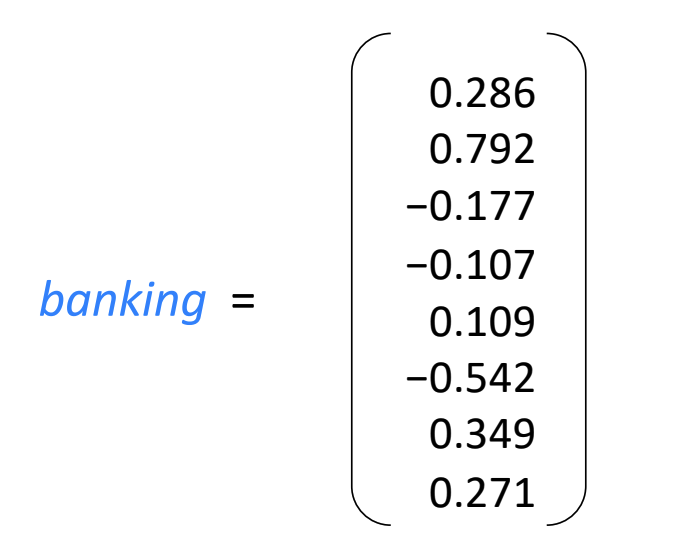Word vector sample from Stanford tutorial https://cdn.hashnode.com/res/hashnode/image/upload/v1616661445528/GuBVj_XG0.html

There are a number of techniques for Word Vectorization to convert text into integers or vectors to which mathematical operations can be applied in order to extract insights from the given data.

## Rewind To Pre-2013 Era Of NLP

### Denotational Semantics

The idea in denotational semantics is represented by word meaning which is the same way as a Thesaurus works. The best available solution is **WordNet **which contains synonym sets

``````>>> dog.lch_similarity(cat)  # doctest: +ELLIPSIS
2.028...

>>> hit.lch_similarity(slap)  # doctest: +ELLIPSIS
1.312...

>>> wn.lch_similarity(hit, slap)  # doctest: +ELLIPSIS
1.312...

>>> print(hit.lch_similarity(slap, simulate_root=False))
None

>>> print(wn.lch_similarity(hit, slap, simulate_root=False))
``````

However, there were a lot of shortcomings for this

• Great as a resource but missing nuance

• Missing new meanings of words like lol, whatsup, etc

• Requires human labor to create and adapt

• Not that accurate

### One-hot Vectors

These basically were kind of a foundation for building Word Vectorization. Traditional NLP had words referred to as localist representations. In a traditional process, if we wanted to train an ML model, we had to come up with categorical structures similar to building a classification model.

These one-hot encoded vectors should look something similar like this, however, they would be way bigger in size, equivalent to the size of the vocabulary

``````motel = [0 0 0 0 0 0 0 0 0 0 1 0 0 0 0]
hotel = [0 0 0 0 0 0 0 1 0 0 0 0 0 0 0]
``````

Now the major problem is that languages have a lot of words, infinite space of words, which means the one-hot encoded vectors become really big.

A bigger challenge is that there is no natural notion of similarity for one-hot vectors. Instead of using synonyms and trying relationship similarity tables, another method called Distributional Semantics makes it easier to explore words as vectors. This approach looks at the meaning of the word by looking at the context. For example, the word ‘banking’. Distributional Semantics gives a context to the word where it is used. It also does an excellent job of capturing meaning. For this approach, we still represent the meaning of a word as a numeric vector. The dimension of each word can land up to 50–300–2000–4000. However, this is way smaller than a one-hot vector of 500,000 in traditional NLP.

## Introducing Word2Vec

In 2013 Tomas Mikolov released the paper on an effective way to represent words in the form of word vectors.

Distributed representations of words in a vector space help learning algorithms to achieve better performance in natural language processing tasks by grouping similar words. Mikolov introduced the Skip-gram model, an efficient method for learning high-quality vector representations of words from large amounts of unstructured text data. Unlike most of the previously used neural network architectures for learning word vectors, training of the Skip-gram model does not involve dense matrix multiplications. This makes the training extremely efficient: an optimized single-machine implementation can train on more than 100 billion words in one day.

### How does this work?

The word vectorization is mainly based on the idea of representing words by their context. A word’s meaning is given by the words that frequently appear close-by.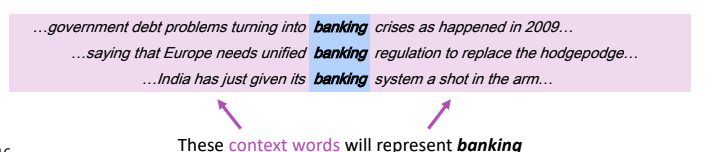Word vector sample from Stanford tutorial https://youtu.be/8rXD5-xhemo

• Use a large corpus of text — this can be wikepedia or any other text in large amount

• Every word in a fixed vocabulary is represented by a vector

• Go through each position t in the text, which has a center word c and context (“outside”) words o

• Use the similarity of the word vectors for c and o to calculate the probability of o given c (or vice versa)

• Keep adjusting the word vectors to maximize this probability

Calculation of word vector will somewhat look like this where we approach center word one by one and look at its context words bounded by a window length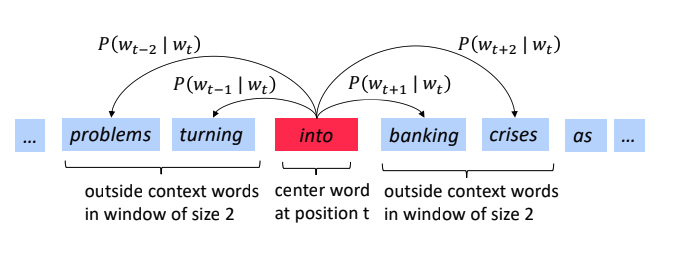Word vector sample from Stanford tutorial https://youtu.be/8rXD5-xhemo

So for each position= 1 to T we predict context words within a window of fixed size m, given center word Wj we calculate the likelihood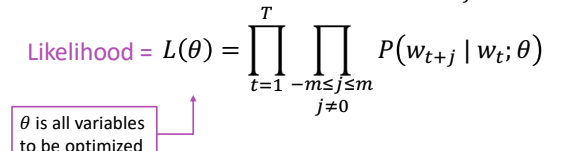Word vector equation from Stanford tutorial https://youtu.be/8rXD5-xhemo

The objective function J is the (average) negative log likelihood — minimizing this will result in effective vectors

Minimizing objective function ⟺ Maximizing predictive accuracy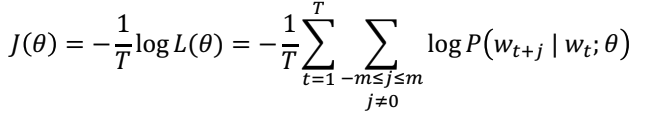Word vector equation from Stanford tutorial https://youtu.be/8rXD5-xhemo

The end result of this calculation should look somewhat similar like the image shown below where similar vectors are closer to each other in the word vector space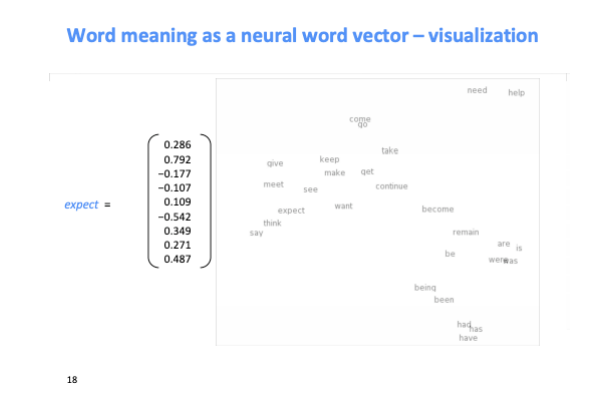Word vector equation from Stanford tutorial https://youtu.be/8rXD5-xhemo

If you look at the figure above, we have words with their vector representations. Since each is going to have a vector representation, we have a vector space in which we can place all of the words. Now, this is a very big space, built from 100 dimension word vectors. It is very hard to visualize 100 dimensional word vectors that are being projected down to a 2D space. While this can represent what is in original space, even 2D space somehow shows related words are close.

## How Word Vectorization Helps In Deep Learning

Word Vectorization helps in use cases including computing similar words, text classifications, document clustering or grouping, feature extraction for text classifications, and natural language processing (NLP).

Now there are various ways to convert sentences into vectors. The pre-trained methods include:

1. Word2Vec from Google is an iterative updating algorithm that learns the vector representation of words, in some sense captures their meaning, maximises objective function by putting similar words in nearby space.

2. Fasttext from Facebook is an extension of the Word2Vec model and instead of learning vectors for words directly, it represents each word as an n-gram of characters. Once the word has been represented using character n-grams, a skip-gram model is trained to learn the embeddings. It works well with rare words.

3. GloVe from Stanford allows converting files of GloVe vectors into Word2Vec. Unlike Facebook and Google’s word vectors which have a large vocabulary and a lot of dimensions, GloVe is small with 100 dimensions and 50 dimensions.

Post 2013, Neural Net Style representation of NLP came into being, making word vectorization a simple and scalable solution. Word2Vec has had the biggest impact on NLP with deep learning. It is a framework for learning vectors. This approach lets you start with a big pile of text — web pages, news article, “corpus” body of text. The idea behind Word2Vec is when we have a large corpus of text, every word in a fixed vocabulary is represented by a vector. You go through each position t in the text, which has a center word c, and context (“outside”) words o. Use the similarity of the word vectors for c and o to calculate the probability of o given c (or vice versa). Keep adjusting the word vectors to maximize this probability. We can repeat this a billion times (iteration) to build a word vector space.